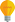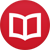# Integral of $\cot(x)$

by Jakub MarianTip: See my list of theMost Common Mistakes in English. It will teach you how to avoid mis­takes with com­mas, pre­pos­i­tions, ir­reg­u­lar verbs, and much more.

To integrate $\cot(x)$, recall that

$$\cot(x) = \frac{\cos(x)}{\sin(x)}\,,$$

so

$$∫ \cot(x)\,dx = ∫ \frac{\cos(x)}{\sin(x)}\,dx\,.$$ By choosing $u = \sin(x)$, that is, “$du = \cos(x)\,dx$” (in quotation marks because this expression does not make sense mathematically, but it does work formally), we get $$∫ \frac{\cos(x)}{\sin(x)}\,dx = ∫ \frac{1}{u}\,du = \log(u)+C = \log(\sin(x))+C$$

By the way, I have written several educational ebooks. If you get a copy, you can learn new things and support this website at the same time—why don’t you check them out?